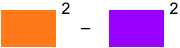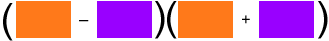James Secondary home school 12th grade Student I cannot figure these out I was wondering if you could help me? I have no one to answer my questions. (7x2 – 3yz)2 – (7x2 + 3yz)2 Use Pascal’s triangle to expand (2x – y)4 8x3 y - x3 y4 (m + 3n)2 – 144 12x4 y – 16x3 y2 – 60x2 y3 p3 q2 – 9p3 + 27q2 – 243 Hi James, A significant part of algebra is "pattern recognition". By that I mean the ability to look at an expression and see some underlying pattern. For example in problem 1 what I see iswhich is a difference of squares and factors asIn other words, a2 - b2 = (a - b)(a + b) Hence (7x2 – 3yz)2 – (7x2 + 3yz)2 = [(7x2 – 3yz) – (7x2 + 3yz)][(7x2 – 3yz) + (7x2 + 3yz)] Now simplify. For probmem 3 there is an x and a y in each of the two terms. Hence x3y is a common factor and you can write 8x3 y - x3 y4 = x3y(8 - y3) Since 8 = 23 this becomes 8x3 y - x3 y4 = x3y(23 - y3) The pattern to recognize this time is that 23 - y3 is a difference of cubes that you can factor. Now try problems 4, 5 and 6. You need to look for common factors and "patterns". Cheers, Penny Go to Math Central